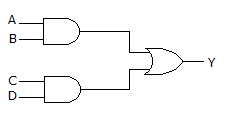# Electronics and Communication Engineering - Digital Electronics

36.

A 4 bit down counter can count from

 A. 0000 to 1111 B. 1111 to 0000 C. 000 to 111 D. 111 to 000

Explanation:

It can count from 15 to 0 or 1111 to 0000.

37.

Assertion (A): In computers the data is stored in hexadecimal form

Reason (R): Hexadecimal representation is short as compared to binary representation.

 A. Both A and R are correct and R is correct explanation of A B. Both A and R are correct but R is not correct explanation of A C. A is true, R is false D. A is false, R is true

Explanation:

Data is stored in binary form only.

38.

For the circuit of the given figure, the output equation isA. Y = ABCD B. Y = AB + CD C. Y = A + BCD D. Y = ABC + D

Explanation:

The inputs A and B are ANDed. Inputs B and C' so ANDed. The outputs of two AND gates are ORed.

39.

Using 2's complement, the largest positive and negative number which can be stored with 8 bits are

 A. +128 and -127 B. +128 and -128 C. +127 and -128 D. +127 and -127

Explanation:

Largest positive number is 0111 111 = + 127 and largest negative number is 1000 0000 = -128.

40.

Three Mod-16 counters are connected in cascade. The maximum counting range is

 A. 16 B. 256 C. 4096 D. none of the above